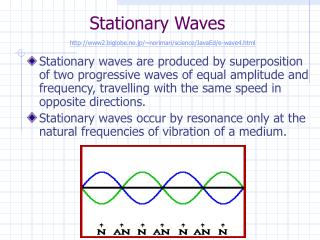DownloadDownload PresentationStationary Waves

# Stationary Waves

Télécharger la présentation## Stationary Waves

- - - - - - - - - - - - - - - - - - - - - - - - - - - E N D - - - - - - - - - - - - - - - - - - - - - - - - - - -
##### Presentation Transcript

1. Stationary Waves http://www2.biglobe.ne.jp/~norimari/science/JavaEd/e-wave4.html • Stationary waves are produced by superposition of two progressive waves of equal amplitude and frequency, travelling with the same speed in opposite directions. • Stationary waves occur by resonance only at the natural frequencies of vibration of a medium.

2. Nodes Antinodes λ Properties of a stationary wave (1) • Stationary waves have nodes where there is no displacement at any time. • In between the nodes are positions called antinodes, where the displacement has maximum amplitude.

3. Properties of a stationary wave (2) • All particles between two adjacent nodes are in phase. • The waveform in a stationary wave does not move through medium; energy is not carried away from the source. • The amplitude of a stationary wave varies from zero at a node to maximum at an antinode, and depends on position along the wave.

4. Production of Stationary Waves http://www2.biglobe.ne.jp/~norimari/science/JavaEd/e-wave5.html • A stationary wave would be set up by causing the string to oscillate rapidly at a particular frequency. • If the signal frequency is increased further, overtone patterns appear.

5. Resonant Frequencies of a Vibrating String • From the experiment, we find that • There is a number of resonant frequencies in a vibrating string, • The lowest resonant frequency is called the fundamental frequency (1st harmonic), • The other frequencies are called overtones (2nd harmonic, 3rd harmonic etc.), • Each of the overtones has a frequency which is a whole-number multiple of the frequency of the fundamental.

6. Factors that determine the fundamental frequency of a vibrating string • The frequency of vibration depends on • the mass per unit length of the string, • the tension in the string and, • the length of the string. • The fundamental frequency is given by where T = tension  = mass per unit length L = length of string

7. Picture of Standing Wave Name Structure Modes of vibration of strings http://id.mind.net/~zona/mstm/physics/waves/standingWaves/standingWaves1/StandingWaves1.html 1st Harmonic or Fundamental 1 Antinode 2 Nodes L = ½λ1 f1 = v/2L L = λ2 f2 = v/L 2nd Harmonic or 1st Overtone 2 Antinodes 3 Nodes 3rd Harmonic or 2nd Overtone 3Antinodes 4 Nodes L = 1½λ3 f3 = 3v/2L 4th Harmonic or 3rd Overtone 4Antinodes 5 Nodes L = 2λ4 f4 = 2v/L 5th Harmonic or 4th Overtone 5Antinodes 6 Nodes L = 2½λ5 f5 = 5v/2L

8. Vibrations in Air Column http://www.walter-fendt.de/ph11e/stlwaves.htm • When a loudspeaker producing sound is placed near the end of a hollow tube, the tube resonates with sound at certain frequencies. • Stationary waves are set up inside the tube because of the superposition of the incident wave and the reflected wave travelling in opposite directions.

9. Factors that determine the fundamental frequency of a vibrating air column • The natural frequency of a wind instrument is dependent upon • The type of the air column, • The length of the air column of the instrument. Open tube Closed tube

10. Picture of Standing Wave Name Structure Modes of vibration for an open tube 1st Harmonic or Fundamental 2 Antinodes 1 Node L = ½λ1 f1 = v/2L 2nd Harmonic or 1st Overtone 3 Antinodes 2 Nodes L = λ2 f2 = v/L L = 1½λ3 f3 = 3v/2L 3rd Harmonic or 2nd Overtone 4 Antinodes 3 Nodes 4th Harmonic or 3rd Overtone 5 Antinodes 4 Nodes L = 2λ4 f4 =2v/L L = 2½λ5 f5 = 5v/2L 5th Harmonic or 4th Overtone 6 Antinodes 5 Nodes

11. Picture of Standing Wave Name Structure Modes of vibration for a closed tube 1st Harmonic or Fundamental 1 Antinode 1 Node L = ¼λ1 f1 = v/4L 3rd Harmonic or 1st Overtone 2 Antinodes 2 Nodes L = ¾λ3 f3 =3v/4L 5th Harmonic or 2nd Overtone 3 Antinodes 3 Nodes L = 1¼λ5 f5 =5v/4L 7th Harmonic or 3rd Overtone 4 Antinodes 4 Nodes L = 1¾λ7 f7 = 7v/4L 9th Harmonic or 4th Overtone 5 Antinodes 5 Nodes L = 2¼λ9 f9 =9v/4L

12. resultant Fundamental 1st overtone 2nd overtone 3rd overtone The quality of sound (Timbre) http://surendranath.tripod.com/Harmonics/Harmonics.html • The quality of sound is determined by the following factors: • The particular harmonics present in addition to the fundamental vibration, • The relative amplitude of each harmonic, • The transient sounds produced when the vibration is started.

13. Reflector Detector Wave source Investigating stationary waves using sound waves and microwaves • Moving the detector along the line between the wave source and the reflector enables alternating points of high and low signal intensity to be found. These are the antinodes and nodes of the stationary waves. • The distance between successive nodes or antinodes can be measured, and corresponds to half the wavelength λ. • If the frequency f of the source is known, the speed of the two progressive waves which produce the stationary wave can be obtained.

14. Chladni’s Plate • Chladni’s plate is an example of resonance in a plate. • There are a number of frequencies at which the plate resonate. Each gives a different pattern.